# 3 Easy Tricks to Create New Columns in Python Pandas

ModelingPythonTools & Languagesposted by ODSC Community February 9, 2022

In data processing & cleaning, we need to create new columns based on values in existing columns. In this blog, I...In data processing & cleaning, we need to create new columns based on values in existing columns. In this blog, I explain How to create new columns derived from existing columns” with 3 simple methods.

You can master them in just under 5 minutes to save time in the long run!

Let’s jump in!

If you wish, you can follow along with the dataset, which I created for fun! You can have a look at my Notebook as well (Link at the end).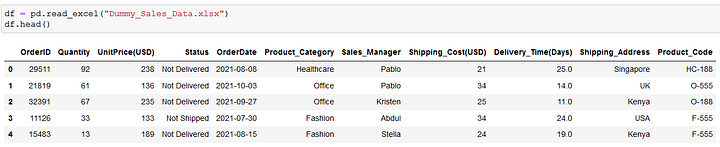Dummy Sales Data | Image by Author

### Use lambda Function with apply() method

The most common way of creating a new column is by doing some operation on the existing column.

Often we need to perform a complex calculation on the existing column and create a new column with the calculated values.

`pandas.DataFrame.apply()` is the solution

For example, let’s create a column Shipment_Size based on the column Quantity in the dataset. The values in this new column should be Small, Medium, and Large depending on the values in the column Quantity.

```def shipsize(row):
if row['Quantity'] > 0 and row['Quantity'] <= 30:
return 'Small'
elif row['Quantity'] > 30 and row['Quantity'] <= 60:
return 'Medium'
elif row['Quantity'] > 60  and row['Quantity'] <= 100:
return 'Large'
return 'NotDefined'```

However, in real-life scenarios, this function can be much more complex.

Then, the new column can be easily created as below

`df['Shipment_Size'] = df.apply(lambda row: shipsize(row), axis=1)`

Putting all steps together, finally, we can see an extra column is added to `df` .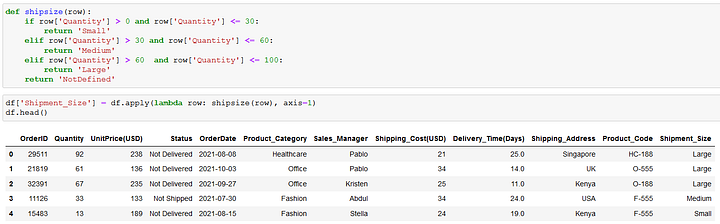Use Lambda function with apply() to create new column | Image by Author

There are many debates about whether to use or not to use the `.apply()` method. Here is an interesting discussion about it on stackoverflow.

Also, if you are interested in knowing how `pandas.DataFrame.apply()` works, then I recommend this in-depth article about it.

### Use NumPy.select() method

Much better and faster performance can be obtained by using the select() method in NumPy.

.select() is 155X faster ⚡ than .apply()

It has a simple syntax, `select(condlist, choicelist)` . And it returns an array drawn from elements in `choicelist`, depending on the condition in `condlist.`

For example, let’s create a column Shipment_Size based on the column Quantity in the dataset. But this time using the`numpy.select()` method.

Let’s start with creating a list of conditions `condlist` and list of choices `choicelist` as below.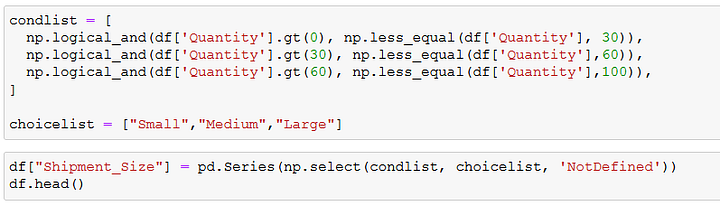condlist and choicelist for numpy.select() | Image by Author

Then, creating a new column is just a one-liner.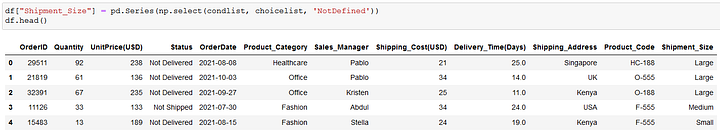Create a new column using numpy.select() | Image by Author

As `numpy.select()` returns an array of data type `numpy.ndarray` , it should be converted in pandas series using `pd.Series` to make a new column.

The official documentation of `numpy.select()` can be found here.

### Use Pandas.DataFrame.loc() method

Lastly, we can also use the `.loc()` method in Pandas DataFrame to create a new column.

This method is quite straightforward and self-explanatory as compared to `.apply()` and `.select()` .

The syntax is quite simple and straightforward.

`Dataframe_name.loc[condition, new_column_name] = new_column_value`

The `new_column_value` is the value assigned in the new column if the `condition` in `.loc()` is True.

For example, let’s create the column Shipment_Size one last time, in this case using `.loc()` as shown below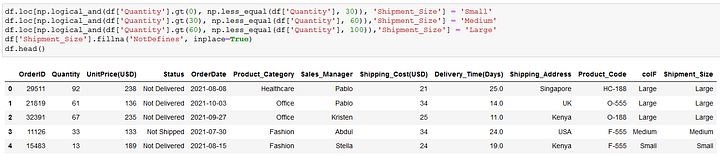Creating new column using pandas.DataFrame.loc() | Image by Author

Although, it is slower than `numpy.select()`, it is still 50 times faster than `pandas.DataFrame.apply()`.

The more details, I recommend reading the interesting article here.

Here is the Notebook with all examples.About Suraj Gurav

Product Manager | Top Writer in AI, Startup, Life | Author | Data Analyst | Systems Engineer | Ex-Bosch | Python | SQL | Power BI | RWTH Aachen Germany## ODSC Community

The Open Data Science community is passionate and diverse, and we always welcome contributions from data science professionals! All of the articles under this profile are from our community, with individual authors mentioned in the text itself.

1